# QOTD - Quantitative Aptitude Problem - Mensuration

2019-08-22 | Team PendulumEdu

A rhombus is inscribed in a circle in such a way that one vertex of rhombus is on the center while other three vertices are on the circumference of the circle. If the radius of the circle is 4 m then, what will be the ratio of area of circle and rhombus?

Options: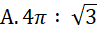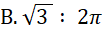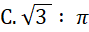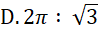Solution:

According to the information given in the question, the schematic will beIn the above figure,

OA = OC = OB = radius of circle

Since, the opposite sides of a rhombus are equal

Thus, OA = CB and OC = AB

So, from the above figure,

Area of rhombus OABC (AR) will be = area of triangle OAB + area of triangle OBC

Since, both are equilateral triangle, the area will be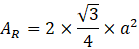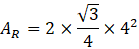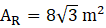Now, area of circle Ac will be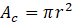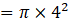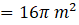Therefore, the ratio of the area of circle to that of rhombus will beHence, (D) is the correct answer.

Such type of question is asked in exams like SSC CGL, SSC MTS, SSC CPO, SSC CHSL, RRB JE, RRB NTPC, RRB GROUP D, RRB OFFICER SCALE-I, IBPS PO, IBPS SO, RRB Office Assistant, IBPS Clerk, RBI Assistant, IBPS RRB OFFICER SCALE 2 & 3. Try and attempt free mock tests at PendulumEdu and learn new techniques to solve such questions in less time. One can also find help and support through PendulumEdu’s one-to-one session where the experts will clear all exam related doubts.0